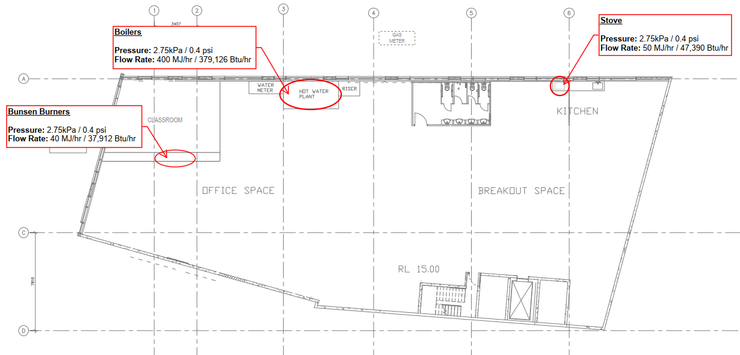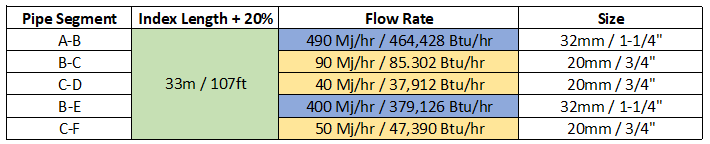•H2X Team

# How to Size Gas Pipework in 4 Simple Steps

Introduction – Understand the principles

As gas goes through a pipe, pressure loss is caused by friction between the gas and the pipe walls.

The bigger the pipe, the less pressure loss. This can be visualised below:When sizing gas pipework, the pressure loss through the pipes cannot exceed the difference between the starting pressure and the pressure required at the appliances.

Based on the example image above, if you need 2.75 kPa / 0.4 psi at the appliance:

• The 50mm / 2" pipe is too small

• The 100mm / 4" pipe is sufficient

• The 150mm / 6" pipe is sufficient but unnecessarily large (not cost effective)

Now lets work through a simple example project together.

Step 1 – Where are the gas requirements?

Find out from the project team which appliances require gas.

In this example we have stoves in a kitchen, bunsen burners in a classroom, and boilers in the plant room.Step 2 – What are the requirements?

You can find out the requirements by asking the relevant project team member or looking up the technical data of the appliance. The information you need to know is the flow rate of the appliance and the inlet pressure.

In this example, all appliances have differing flow rates with the same inlet pressure of 2.75 kPa / 0.4 psi.Step 3 - Draw the pipe layout

In the most efficient way possible, draw the pipe layout from the gas meter to the appliances (minimise the amount of pipe and fittings).Step 4 – Size the pipes

First you need to determine the index length. The index length is the route from the gas meter to the furthest appliance.

As you can see in the example below, the stove in the kitchen is the furthest away appliance, and is therefore the index length (highlighted in yellow).The index length from A-F is 27m / 88 ft. It is common practice to add 20% on the index length to cater for pressure loss through bends and fittings. This makes the index length 33m / 108 ft.

From here we can create a table to record the pipe sizes for each segment. The process to follow for each pipe segment is:

• Always use the index length; and

• Use the combined flow rate of the downstream appliances.Now we can use a spreadsheet or pre-calculated tables to fill in the 'size' column on the table.

Using the pre calculated table below, the index length is rounded up and shown in green. The pipe segments B-C, C-D, and C-F are shown in yellow, B-E and A-B is shown in blue.

How do I read the below table?

1. Find the length of pipe that is equal to or greater than the index length; then

2. Make your way through the below numbers until you find the first one that is equal to or greater than the flow rate; then

3. Look horizontally to the left to find what the corresponding pipe size is.Now we can complete the table with the results.Then the last step is to show the pipe sizes on the drawing.Other items that you will need to consider on more complex projects are:

• Diversity – if you have lots of appliances, they will not operate simultaneously so you do not need to allow for 100% demand

• Minimum pressure - you may have appliances that have different maximum pressure requirements, in this case it would be best to use the most onerous

• Height – if you have a very tall building, the height will affect your calculations

A better way to do it

The video below shows a better way to size a gas system using H2X’s design and calculation software. Not only does it automatically provide the results as you draw out the system, it is flexible to suit future design changes, you automatically get the cost of the system, it is easy to review, and you get a clear digital markup that you can share with the project team.

You can start a free trial of H2X today.

###### FEATURES

Water Services Calculations

Gas Services Calculations

Heating Calculations

Sanitary Drainage Calculations

3D Revit Model

Cost & Bill of Materials Export

Design Warnings